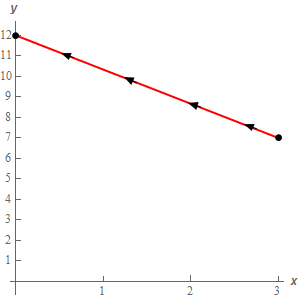Paul's Online Notes
Home / Calculus III / Line Integrals / Line Integrals of Vector Fields
Show Mobile Notice Show All Notes Hide All Notes
Mobile Notice
You appear to be on a device with a "narrow" screen width (i.e. you are probably on a mobile phone). Due to the nature of the mathematics on this site it is best views in landscape mode. If your device is not in landscape mode many of the equations will run off the side of your device (should be able to scroll to see them) and some of the menu items will be cut off due to the narrow screen width.

### Section 16.4 : Line Integrals of Vector Fields

1. Evaluate $$\displaystyle \int\limits_{C}{{\vec F\centerdot d\vec r}}$$ where $$\vec F\left( {x,y} \right) = {y^2}\,\vec i + \left( {3x - 6y} \right)\vec j$$ and $$C$$ is the line segment from $$\left( {3,7} \right)$$ to $$\left( {0,12} \right)$$.

Show All Steps Hide All Steps

Start Solution

Here is a quick sketch of $$C$$ with the direction specified in the problem statement shown.Show Step 2

Next, we need to parameterize the curve.

$\vec r\left( t \right) = \left( {1 - t} \right)\left\langle {3,7} \right\rangle + t\left\langle {0,12} \right\rangle = \left\langle {3 - 3t,7 + 5t} \right\rangle \,\,\,\,\,\,\,\,0 \le t \le 1$ Show Step 3

In order to evaluate this line integral we’ll need the dot product of the vector field (evaluated at the along the curve) and the derivative of the parameterization.

Here is the vector field evaluated along the curve (i.e. plug in $$x$$ and $$y$$ from the parameterization into the vector field).

$\vec F\left( {\vec r\left( t \right)} \right) = {\left( {7 + 5t} \right)^2}\,\vec i + \left( {3\left( {3 - 3t} \right) - 6\left( {7 + 5t} \right)} \right)\vec j = {\left( {7 + 5t} \right)^2}\,\vec i + \left( { - 33 - 39t} \right)\vec j$

The derivative of the parameterization is,

$\vec r'\left( t \right) = \left\langle { - 3,5} \right\rangle$

Finally, the dot product of the vector field and the derivative of the parameterization.

$\vec F\left( {\vec r\left( t \right)} \right)\centerdot \vec r'\left( t \right) = - 3{\left( {7 + 5t} \right)^2} - 5\left( {33 + 39t} \right)$ Show Step 4

Now all we need to do is evaluate the integral.

\begin{align*}\int\limits_{C}{{\vec F\centerdot d\vec r}} & = \int_{0}^{1}{{ - 3{{\left( {7 + 5t} \right)}^2} - 5\left( {33 + 39t} \right)\,dt}}\\ & = \left. {\left[ { - \frac{1}{5}{{\left( {7 + 5t} \right)}^3} - 165t - \frac{{195}}{2}{t^2}} \right]} \right|_0^1 = \require{bbox} \bbox[2pt,border:1px solid black]{{ - \frac{{1079}}{2}}}\end{align*}